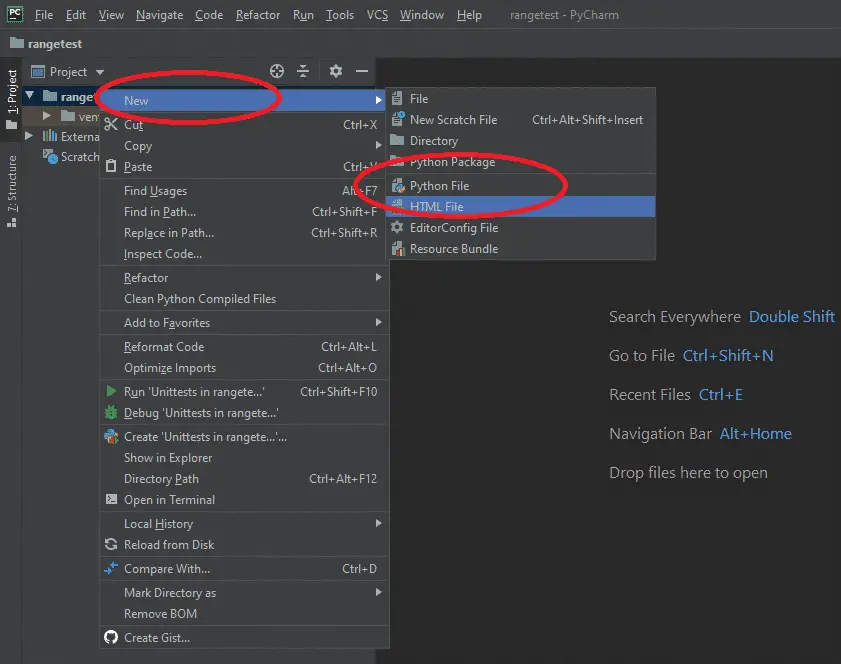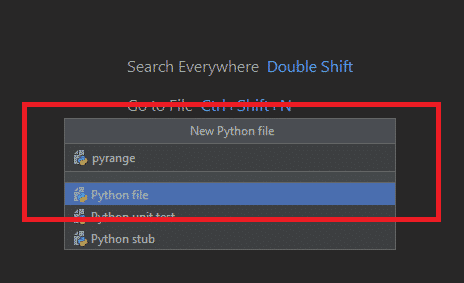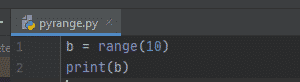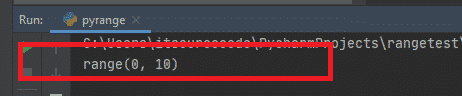# Range Function In Python Explained with Examples 2022

In this tutorial, I will teach you about what is Range Function in Python and How to use it using different Example.

So to start our journey, let us visit first some of the frequently asked questions about range() in python.

Watch the video tutorial here before you proceed.

What Is Range In Python?

The range() built-in function in python is used to returns a series or sequence of numbers starting with an index of zero(0) by default and Incremented by one(1) by default and will ends at a specified value or number.

How to use Range in Python?

To use range in python, you need to use For loop so that you can iterate over the sequence of numbers produced by the range.

What is the difference between range() and xrange() functions?

The range is the renamed version of xrange in Python(2.x).

At this time, we will continue further with our discussion using different example on how to use range in python.

## Syntax of range() function and it’s arguments

`range(start, stop, step)`

Since have already learned the basic ideas about range() in python. At this point, we will now proceed how to use range in python using the actual coding.

For this tutorial, I am using python 3.8.2 version. you can download it in python.org website. link and for this demonstration the Pycharm IDE.

Range Function in Python

• Step 1: Open Pycharm IDE.

When the Pycharm is open, you need to create a Project and name it as “rangetest”.

• Step 2: Create Python file

Right Click Project, click “new” and Select “Python File“.• Step 3: Create Python File

On the new python file, type “pyrange” as the name of this python file and press Enter.• Step 4: Start Coding

To start the coding of range() in python. add the simple code.• Step 5: Run Project

To run python project, you can press “Shift + F10” or simply click the Play button.

• Step 6: Check the result

As expected, when you successfully run the project, the result will looks like as shown below.## Range() Function with Stop Parameter

Sample Code:

``````# Program to explain range() function
# range() function with single parameter

# for loop to print number from 0 to 4
for i in range(5):
print(i)``````

Result:

``````0
1
2
3
4``````

Explanation:

This is a program that will print the numbers 0 through 4. In this case, the range() method is used with a for loop. The numbers in the range are looped through using the variable i.

There is no other parameter in the line range(5). So, this is the stop parameter for the range() method. Because of this, the result is 0,1,2,3,4.

So, since the stop parameter is 5, the range() method stops printing at 4. Also, if you don’t give a start parameter, range() uses 0 as the default parameter.

## 3 Variant of using python range function

At this time, we will now explore more examples of using the three variance of range function in python.

### Range with One Argument

For the first variant, we will use one Argument:

Sample code:

```b = range(10)
for a in b:
print("Current value of a: ", a)```

Results:

```Current value of a: 0
Current value of a: 1
Current value of a: 2
Current value of a: 3
Current value of a: 4
Current value of a: 5
Current value of a: 6
Current value of a: 7
Current value of a: 8
Current value of a: 9```

Code Explanation:

In the above code, when the program executes the range() function with one argument. It creates a sequence of numbers from 0 – 9, then it prints the result with each item in the sequence.

Take note:

The range with one argument always start with 0 with an incremental step of 1.(start = 0 and step = 1)

See the Diagram Below:

### Range with Two Arguments

Sample code of using range() function with two arguments:

```b = range(2, 10)
for a in b:
print("Current value of a: ", a)```

Results:

```Current value of a: 2
Current value of a: 3
Current value of a: 4
Current value of a: 5
Current value of a: 6
Current value of a: 7
Current value of a: 8
Current value of a: 9```

Code Explanation:

In the example using range with two arguments which are the (start and stop). The program doesn’t have to start with 0 all the time, just like in our example that the start value is 2. Then it prints the result with each item in the sequence.

It means the we can use this `range()` function in python to generate a series of numbers from X to Y using a range(X, Y).

See the Diagram Below:

### Range with Three Arguments

Sample code of using range() function with three arguments:

```b = range(2, 10, 2)
for a in b:
print("Current value of a: ", a)```

Results

```Current value of a: 2
Current value of a: 4
Current value of a: 6
Current value of a: 8```

Code Explanation:

In the example using range with three arguments which are the (start, stop, step). This time we can select not only where the series of numbers will start and stop, but we can also specify the difference between each number and in our case, the step value is 2.

See the Diagram Below: